# ISEE Middle Level Math : Algebraic Concepts

## Example Questions

### Example Question #1232 : Hspt Mathematics

Simplify: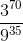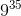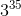Explanation:

To simplify, we want to get the same base in both the numerator and the denominator. This will allow us to easily manipulate the exponents.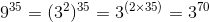Replace the denominator with this value and simplify.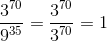### Example Question #1233 : Hspt Mathematics

Simplify: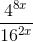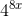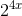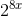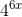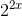Explanation:

To simplify, we want to get the same base in both the numerator and the denominator. This will allow us to easily manipulate the exponents.We can write: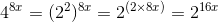and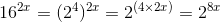Replace the fraction with these values and simplify.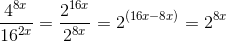### Example Question #1234 : Hspt Mathematics

Which of the following numbers is divisible by 7?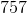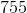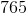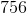Explanation:

The number 756 can be segmented into 700 and 56. Given that 700 is divisble by 7 (700 divided by 7 is 100) and that 56 is divisible by 7 (56 divided by 7 is 8), 756, as the aggregate of 700 and 56, is also divisble by 7.

### Example Question #1235 : Hspt Mathematics

What is the equivalent of this expression?

The square root of 64 divided by the square of 2.Explanation:

The mathematical translation of the square root of 64 divided by the square of 2 is: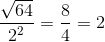### Example Question #1236 : Hspt Mathematics

Which of the following is divisible by 3?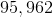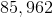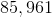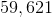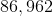Explanation:

A quick trick to remember is that a number is divisible by 3 when the digits of that number add up to a sum that is also divisible by 3.

For example, we know that the number 85,962 must be divisible by 3 because: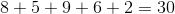30 is divisible by 3; therefore, 85,962 must also be divisible by 3.

In contrast, adding the digits of the other answer choices will give numbers that are not divisible by 3, indicating that the other answer choices are also not divisible by 3.

### Example Question #2201 : Isee Middle Level (Grades 7 8) Mathematics Achievement

Which of the following numbers is divisible by 9?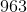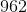None of these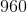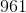Explanation:

A trick to find multiples of 9 is that when the sum of the digits in a number is divisible by 9, then the full number is also divisible by 9.

When the digits of 963 are added together, the sum is 18.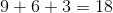18 is divisible by 9, and so the number 963 must also be divisible by 9.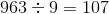### Example Question #2202 : Isee Middle Level (Grades 7 8) Mathematics Achievement

Jack can paint 3 vases in an hour. Jenny can paint 2 vases in an hour. If they work together, how long will it take them to paint 30 vases?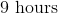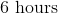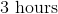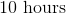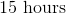Explanation:

If Jack and Jenny paint the vases together, they will paint 5 vases per hour since Jack's rate of painting is 3 vases per hour, while Jenny's rate is 2 vases per hour.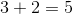If there are 30 vases to paint, and they paint 5 vases per hour, it will take 6 hours to paint all the vases because 30 divided by 5 is 6.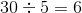### Example Question #2203 : Isee Middle Level (Grades 7 8) Mathematics Achievement

Which of the following is not a factor of 56?

All of these are factors of 56Explanation:

Factors are multiplied together to reach a specific target number. When you divide a target number by one of its factors, the result is always a whole number.

In this case, we can evaluate the numbers that can be multiplied to get 56.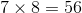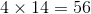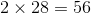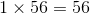12 is not a factor of 56. When you divide 56 by 12, the answer is not a whole number.

### Example Question #2204 : Isee Middle Level (Grades 7 8) Mathematics Achievement

Which of the following is divisible by 13?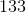None of these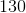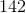Explanation:

When 130 is divided by 13, the result is the whole number, 10.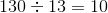130 is the only answer option that results in a whole number when divided by 13, making it the correct answer.

### Example Question #2205 : Isee Middle Level (Grades 7 8) Mathematics Achievement

Jeff has 2 packs of chips. Each pack has 24 chips. If he is going to share all the chips with three friends, so that he and his friends all get an equal number of chips, how many chips does each person get?Explanation:

Given that Jeff has 2 bags, each containing 24 chips, he has a total of 48 chips because 2 times 24 is equal to 48.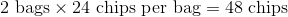There are four total people sharing the chips (Jeff + three friends). We divide the total number of chips by 4 to get our answer.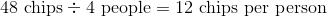### All ISEE Middle Level Math Resources